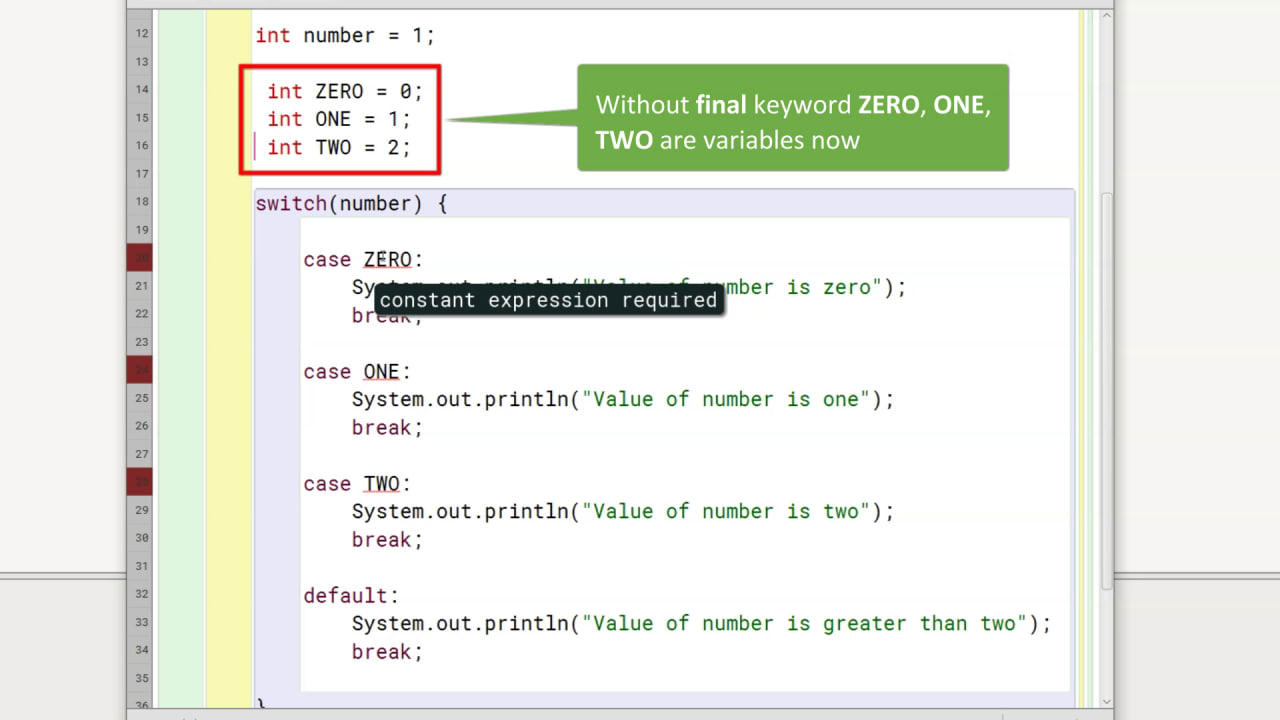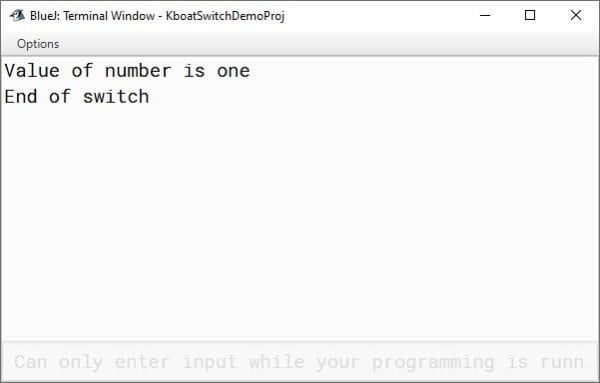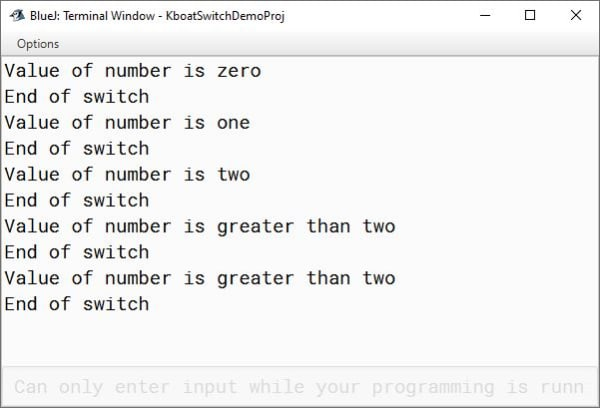# switch Statement

## ICSE Computer Applications

We talked about testing multiple conditions for making a decision while discussing if-else-if ladder. Java provides another control statement, the switch statement for such multi-way branch. It follows this syntax:

```switch (expression){
case value1:
//statement sequence;
break;
case value2:
//statement sequence;
break;
..
..
case valueN:
//statement sequence;
break;
default:
//statement sequence;
break;
}```

Let’s look at a simple example of switch statement and relate it with the syntax:

```/**
* This program shows the usage of switch statement.
*
* @author KnowledgeBoat
* @version 1.0
*/
public class KboatSwitchExample
{
public void demoSwitch() {

int number = 1;

switch(number) {

case 0:
System.out.println("Value of number is zero");
break;

case 1:
System.out.println("Value of number is one");
break;

case 2:
System.out.println("Value of number is two");
break;

default:
System.out.println("Value of number is greater than two");
break;

}

System.out.println("End of switch");

}
}```

The expression of switch statement must resolve to byte, short, int, char, String or an enum. In this example it of type int as the data type of variable `number` is int.

The values specified in the case statements must be unique constant expressions of the same type as the expression in the switch statement.

This point about values of case statements has 3 important things in it. You must understand and remember them. First is that these case values should be unique.

You cannot have duplicate case values within a single switch statement. It will cause an error.

Second is that, case values should be constant expressions. What this means is, the case values can be literals of the same type as the expression in the switch statement. Here, the case values are integer literals `0`, `1`, `2`. They can also be constants declared using the final keyword of the same type as the expression in the switch statement.

So, I can also write the case statements like this.

```public class KboatSwitchExample
{
public void demoSwitch() {

int number = 1;

final int ZERO = 0;
final int ONE = 1;
final int TWO = 2;

switch(number) {

case ZERO:
System.out.println("Value of number is zero");
break;

case ONE:
System.out.println("Value of number is one");
break;

case TWO:
System.out.println("Value of number is two");
break;

default:
System.out.println("Value of number is greater than two");
break;

}

System.out.println("End of switch");

}
}```

This will compile without errors as keyword `final` is making `ZERO`, `ONE`, `TWO` as constants. But they cannot be expressions involving variables like below.Since, I have removed the keyword final, ZERO, ONE, TWO are now variables. There is no guarantee that their values will not change. Values of case statements must be constant expressions. Giving variables as values of case statements results in error.

Coming to the third point, type of each value must be compatible with the type of expression in the switch statement. Here number is of type int and each of the values `0`, `1`, `2` are integer literals so the compiler is happy, and everything works fine. If I change number to String type as shown below, I should get errors for incompatible types.

Now we have some understanding of the syntax of switch statement. Next, let’s try to understand how it works. The switch statement takes the value of the expression and tests it for equality against each of the values specified in the case statements. If it founds a match, then the statements following the case statement are executed. If none of case values match the value of the expression, then the statements in the default case are executed. However, the default case is optional. If no cases match and no default is present, then none of the statements from switch are executed.

The break statements in each of the cases will transfer the program control outside the closing curly brace of the switch statement code block to the line

`System.out.println("End of switch");`

break statements belong to the Jump category of Java’s control statements. They play a very important role in switch statements and loops. We will look at their usage in switch statements in depth just after executing this program. Their usage in loops will be examined in the iteration statements section.

Below is the output of the program. As the value of number is 1, this case statement should get executed.

```case 1:
System.out.println("Value of number is one");
break;```I have introduced a for statement as shown below to execute all the cases. This for loop will test the switch statement with numbers between 0 to 4. Let's examine the output of the program now.

```public class KboatSwitchExample
{
public void demoSwitch() {

//int number = 1;
for (int number = 0; number < 5; number++)
{
switch(number) {

case 0:
System.out.println("Value of number is zero");
break;

case 1:
System.out.println("Value of number is one");
break;

case 2:
System.out.println("Value of number is two");
break;

default:
System.out.println("Value of number is greater than two");
break;

}

System.out.println("End of switch");
}
}
}```As you can see in the output, when value of number is 0, 1 and 2, cases 0, 1 and 2 are executed. For values of number greater than 2 i.e. 3 & 4, default case gets executed.

I hope you have a good understanding of switch statement by now. Next, we will look at the usage of break statements in switch.